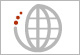Welcome, you are visiting Immersion

Investigating Mathematical Modeling, Experiential Learning and Research
Mathematical modeling, in which mathematics is applied to solve real problems, is a mathematical practice, used by mathematicians and specified in the Common Core State Standards for Mathematics. The need to improve the teaching and learning of mathematics at the elementary level is well documented. Moreover, very limited attention has been paid to how to teach mathematical modeling in the early grades. The importance of this mathematical practice, in which mathematics is applied to real problems, is recognized in the Common Core State Standards for Mathematics and is a foundational tool used by all mathematicians. This project will design and deliver professional development for elementary grades mathematics teachers and will result in curriculum modules that focus on mathematical modeling in the elementary grades. The project is based on mathematical modeling as it is practiced in the workforce and will yield research results regarding how teachers learn about and implement the modeling process.

### Project ContributionsElementary Modeling: Connecting Counting with Sharing
"Examine second-grade students' investigative processes, thinking, and revisions in this lesson using fish crackers. The Counting and Fair-Sharing modeling task highlights several features of the process of mathematical modeling that…Mathematical Modeling: Challenging the Figured Worlds of Elementary Mathematics
"This article is a report on a teacher study group that focused on three elementary teachers' perceptions of mathematical modeling in contrast to typical mathematics instruction. Through the theoretical lens…Engaging Elementary Students in the Creative Process of Mathematizing Their World through Mathematical Modeling
"This paper examines the experiences of two elementary teachers' implementation of mathematical modeling in their classrooms and how the enactment by the teachers and the engagement by students exhibited their…IMMERSION: Mathematical Modeling in the Elementary Grades
Watch Video >> IMMERSION (Integrating Mathematical Modeling, Experiential Learning and Research through a Sustainable Infrastructure and an Online Network) is an NSF-funded STEM-C project that works with elementary grades teachers…New Study Focuses on Mathematical Modeling in the Elementary Grades
"As part of a groundbreaking national study to examine how intensive training can affect elementary school teachers use of mathematical modeling in the classroom, Harvey Mudd College Associate Professor…